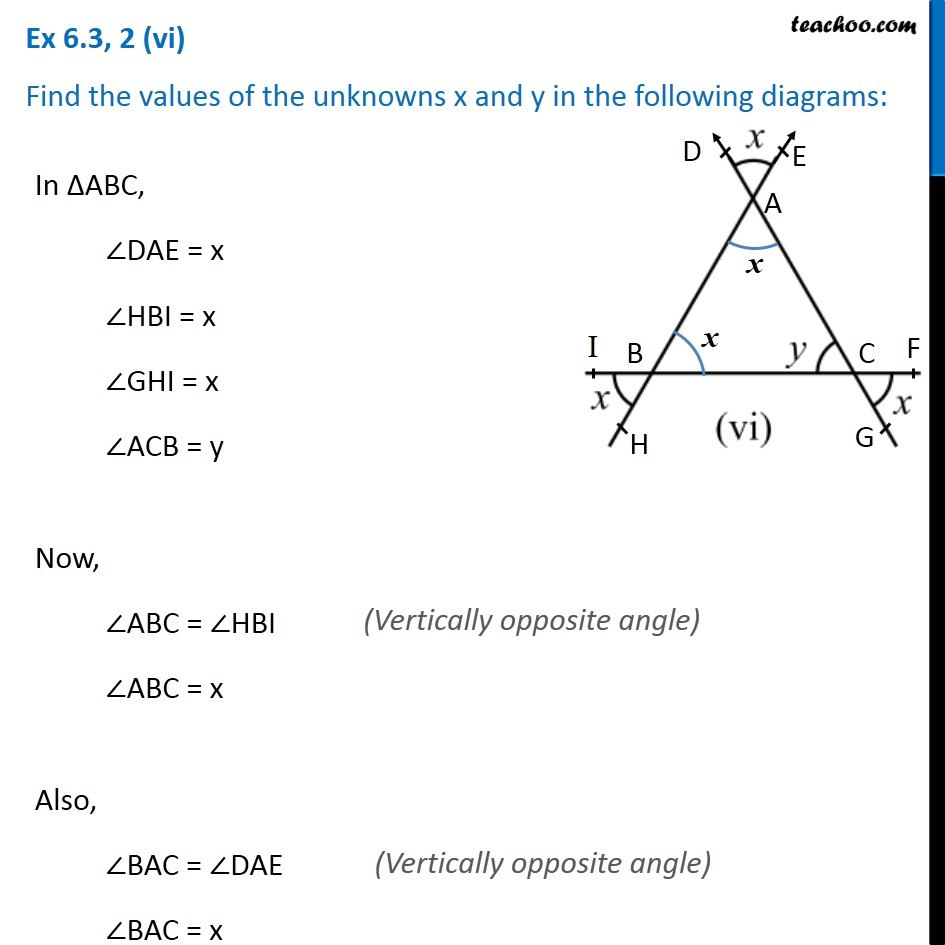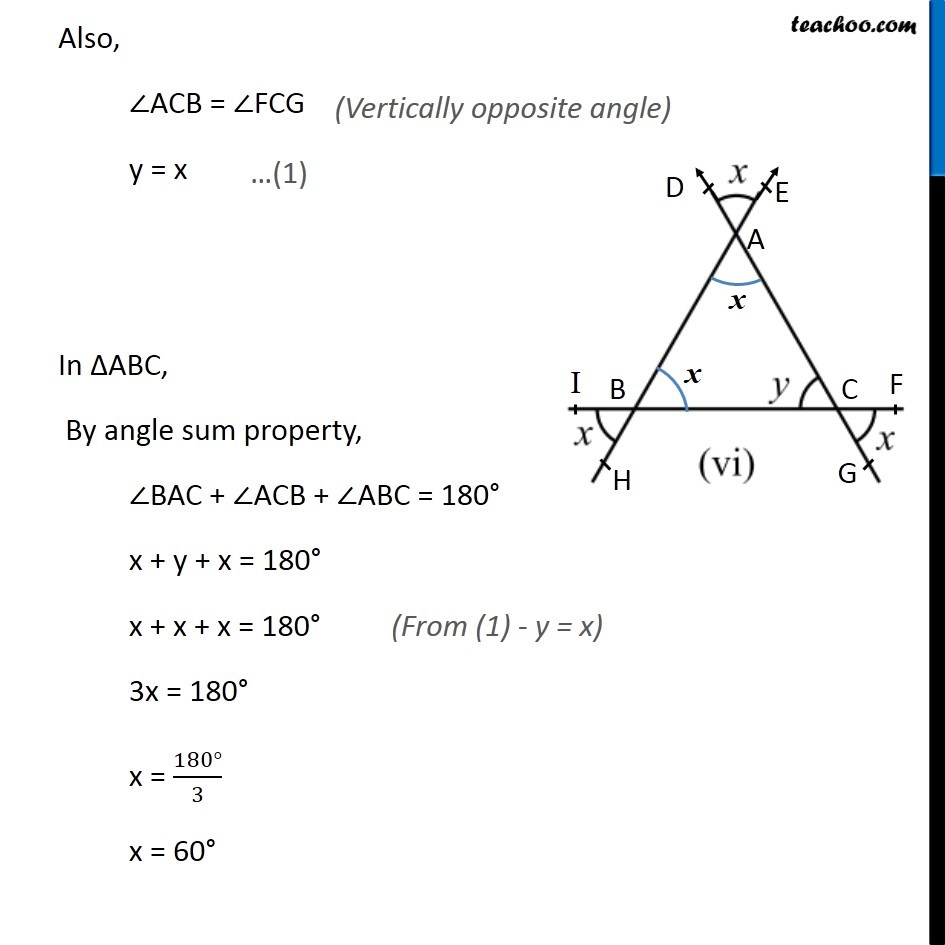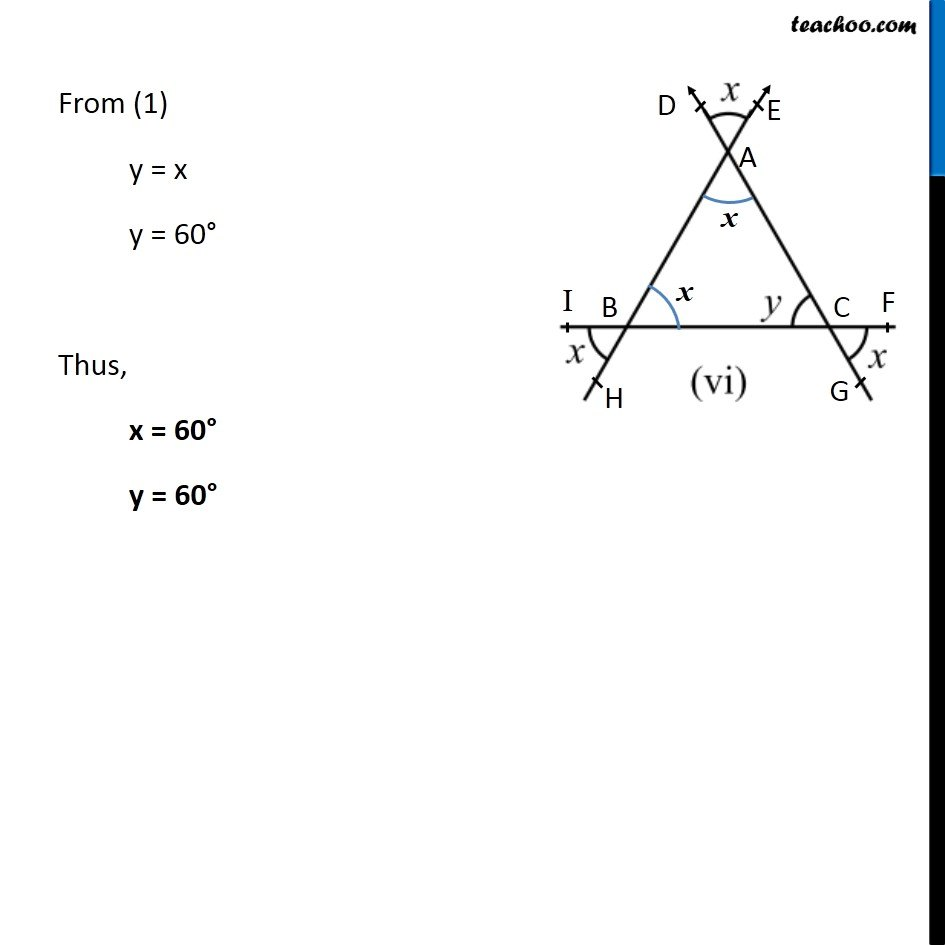Ex 6.3

Chapter 6 Class 7 Triangle and its Properties
Serial order wiseLearn in your speed, with individual attention - Teachoo Maths 1-on-1 Class

### Transcript

Ex 6.3, 2 (vi) Find the values of the unknowns x and y in the following diagrams:In ∆ABC, ∠DAE = x ∠HBI = x ∠GHI = x ∠ACB = y Now, ∠ABC = ∠HBI ∠ABC = x Also, ∠BAC = ∠DAE ∠BAC = x Also, ∠ACB = ∠FCG y = x In ∆ABC, By angle sum property, ∠BAC + ∠ACB + ∠ABC = 180° x + y + x = 180° x + x + x = 180° 3x = 180° x = (180°)/3 x = 60° From (1) y = x y = 60° Thus, x = 60° y = 60°